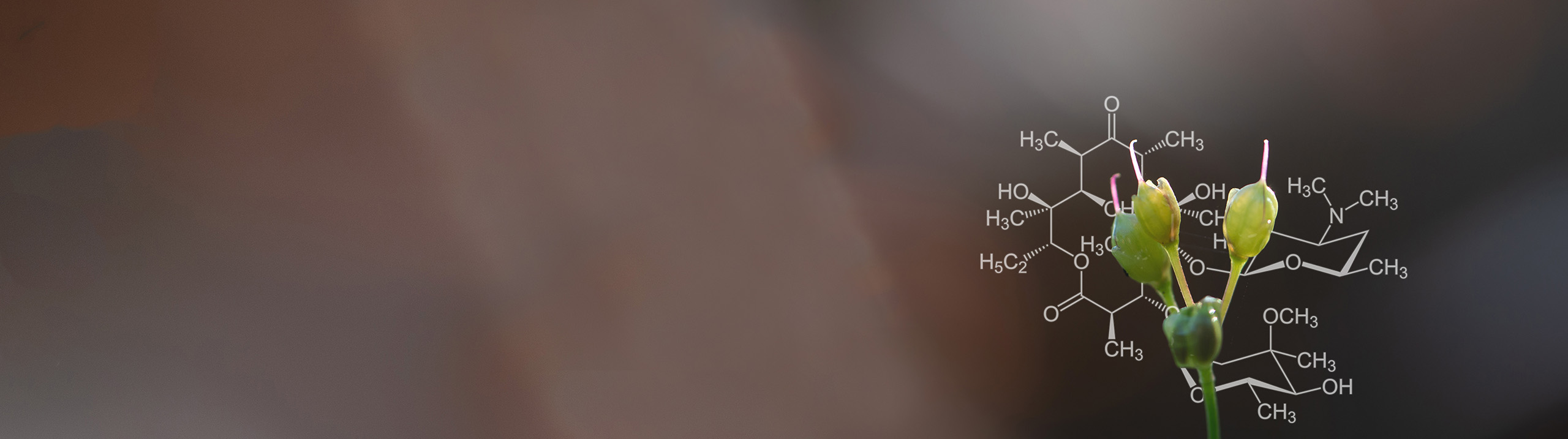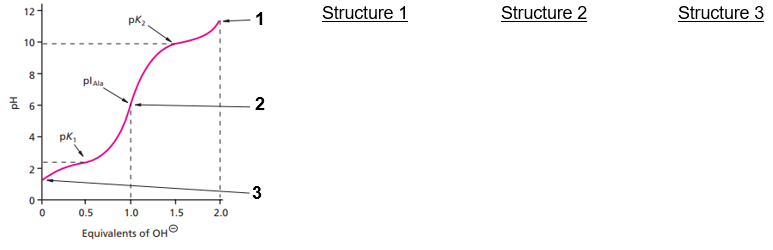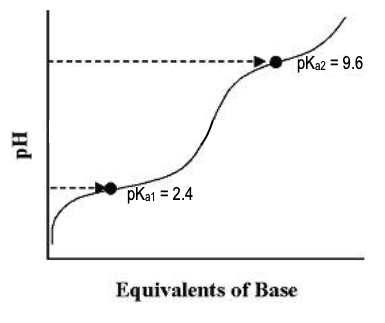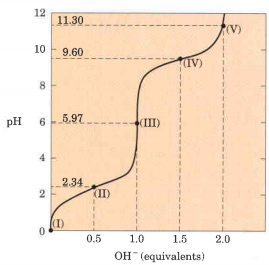Start typing, then use the up and down aroows to select an option from the list.# Biochemistry

Learn the toughest concepts covered in Biochemistry with step-by-step video tutorials and practice problems by world-class tutors

3. Amino Acids

# Titrations of Amino Acids with Non-Ionizable R-Groups

Next Topic1

### concept

Titrations Of Amino Acids With Non-Ionizable R-Groups10m
Play a video:
0
2

### concept

Titrations Of Amino Acids With Non-Ionizable R-Groups13m
Play a video:
0
3
Problem

Draw the predominate structures of Leu at each of the indicated sections ( 1, 2, 3) on its titration curve.4
Problem

Calculate the pI of Ile using its titration curve. Mark the approximate position of the pI on the titration curve.5
Problem

Which of the following compounds would make for the best buffer at pH 8?

6
Problem

Identify the region(s) on glycine’s titration (I, II, III, IV, or V) that corresponds with each statement below:a. Region where Gly predominant species has net charge of +1. ________

b. Region where the average net charge of Gly is +½. ________

c. Region where ½ of Gly’s amino groups are ionized. ________

d. Region where the pH = pKa of carboxyl group. ________

e. Region where the pH = pKa of amino group. ________

f. Regions where Gly has its maximum buffering capacity. _____________

g. Region where the average net charge of Gly is 0. ________

h. Region where Gly’s carboxyl group has been completely titrated. ________

i. Region where Gly has been completely titrated. ________

j. Region where Gly’s predominant species is a zwitterion. ________

k. Region where the average net charge of Gly is -1. ________

l. Region where Gly is a 50:50 mixture of protonated & deprotonated carboxyl group. ________

m. Region indicating Gly’s isoelectric point (pI). ________

n. Region indicating the end of Gly’s titration. ________

o. Regions where Gly has poor buffering power. ____________________